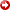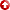[First Hit]# Improving continuity offaultimages using directional smoothing and edge-enhancement filters

## AbstractFaultpicking is a critical, but resource intensive tool for seismic interpretation. Various filters are used in photography and other fields to improve edge detection imaging, the Laplacian of a Gaussian is such a filter. It consists of two phases: the Gaussian operator smoothen high frequency data that would otherwise be exacerbated by the Laplacian, while the latter sharpens the edges. We propose to use a directional Laplacian of a Gaussian operator in order to enhance the image offaultfeatures within dissimilarity data. We start by using a dissimilarity volume from which the covariance matrix is calculated for each sample in it. A set of eigenvalues and eigenvectors are extracted from these matrices, which can be related to the orientation offaultplanesin the data. After that, the data is rotated based on the coordinate system defined by the eigenvectors. Following this rotation it is possible to apply the directional Laplacian of a Gaussian operator to sharpen thefaultimage. In order to remove chaotic data, we apply an eigenvalue-based weight. This weight is applied on the assumption that if the eigenvalues are similar enough then the data is chaotic. The final component of our filter is the cross product of the vectors normal to thefaultplaneswith those defining other structures in the seismic. As a result, only thefaultfeatures will be preserved. So far, discontinuous faults present a challenge for the implementation of this algorithm.